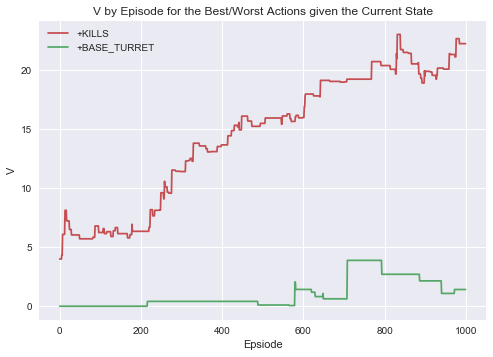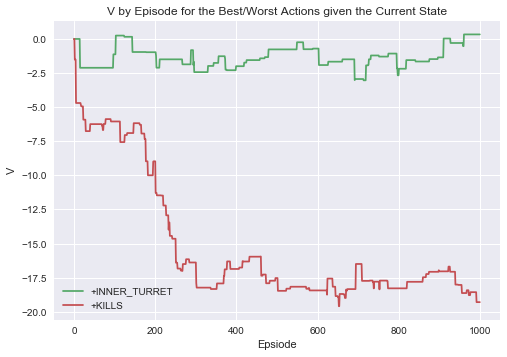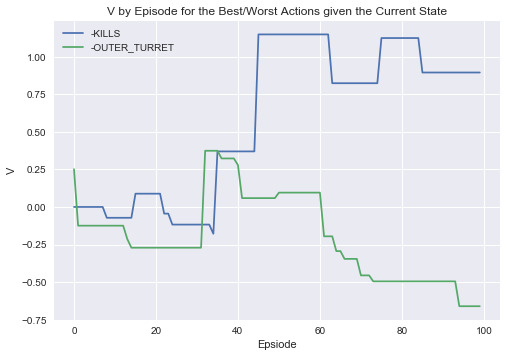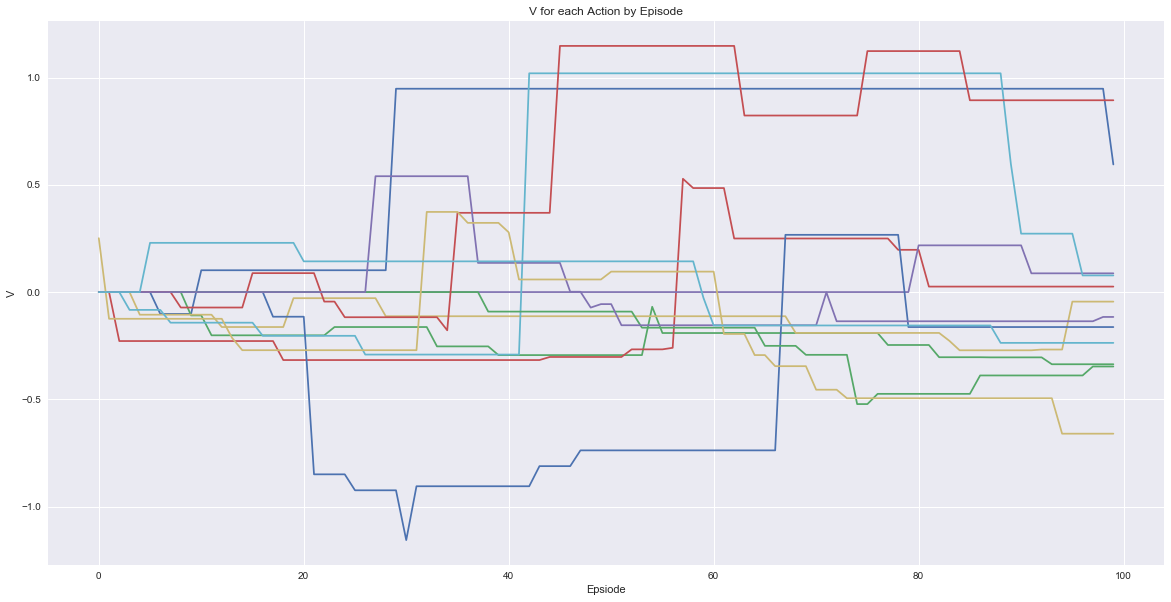LoLModel_Part3

# AI in Video Games: Improving Decision Making in League of Legends using Real Match Statistics and Personal Preferences¶

## Part 3: Introducing Personal Preferences and Concept of Final Output¶

### Motivations and Objectives¶

League of Legends is a team oriented video game where on two team teams (with 5 players in each) compete for objectives and kills. Gaining an advantage enables the players to become stronger (obtain better items and level up faster) than their opponents and, as their advantage increases, the likelihood of winning the game also increases. We therefore have a sequence of events dependent on previous events that lead to one team destroying the other’s base and winning the game.

Sequences like this being modelled statistically is nothing new; for years now researchers have considered how this is applied in sports, such as basketball (https://arxiv.org/pdf/1507.01816.pdf), where a sequence of passing, dribbling and foul plays lead to a team obtaining or losing points. The aim of research such as this one mentioned is to provide more detailed insight beyond a simple box score (number of points or kill gained by player in basketball or video games respectively) and consider how teams perform when modelled as a sequence of events connected in time.

Modelling the events in this way is even more important in games such as League of Legends as taking objectives and kills lead towards both an item and level advantage. For example, a player obtaining the first kill of the game nets them gold that can be used to purchase more powerful items. With this item they are then strong enough to obtain more kills and so on until they can lead their team to a win. Facilitating a lead like this is often referred to as ‘snowballing’ as the players cumulatively gain advantages but often games are not this one sided and objects and team plays are more important.

#### The aim of this is project is simple; can we calculate the next best event given what has occurred previously in the game so that the likelihood of eventually leading to a win increases based on real match statistics?¶

However, there are many factors that lead to a player’s decision making in a game that cannot be easily measured. No how matter how much data collected, the amount of information a player can capture is beyond any that a computer can detect (at least for now!). For example, players may be over or underperforming in this game or may simply have a preference for the way they play (often defined by the types of characters they play). Some players will naturally be more aggressive and look for kills while others will play passively and push for objectives instead.
Therefore, we further develop our model to allow the player to adjust the recommended play on their preferences.

Part 1 and 2 can be found on my Kaggle page (https://www.kaggle.com/osbornep).

### What makes our model ‘Artifical Intellegence’?¶

In out first part, we performed some introductory statistical analysis. For example, we were able to calculate the probability of winning given the team obtains the first and second objective in a match as shown in the image below.

There are two components that make takes our project beyond simple statistics into AI:

• First, the model learns which actions are best with no pre-conceived notion of the game and
• Secondly, it attempts to learn a player’s preference for decisions that will influence the model’s output.

How we define our Markov Decision Process and collect a player’s preference will define what our model learns and therefore outputs.## Pre-Processing and Creating Markov Decision Process from Match Statistics¶

### AI Model II: Introducing Gold Difference¶

I then realised from the results of our first model attempts that we have nothing to take into account the cumulative impact negative and positive events have on the likelihood in later states. In other words, the current MDP probabilities are just as likely to happen whether you are ahead or behind at that point in time. In the game this simply isn’t true; if you are behind then kills, structures and other objectives are much harder to obtain and we need to account for this.
Therefore, we introduce gold difference between the teams as a way to redefine our states. We now aim to have a MDP defining the states to be both the order events occurred but also whether the team is behind, even or ahead in gold. We have categorised the gold difference to the following:

• Even: 0-999 gold difference (0-200 per player avg.)
• Slightly Behind/Ahead: 1,000-2,499 gold difference (200-500 per player avg.)
• Behind/Ahead: 2,500-4,999 gold difference (500-1,000 per player avg.)
• Very Behind/Ahead: 5,000 gold difference (1,000+ per player avg.)
We also now consider no events to be of interest and include this as ‘NONE’ event so that each minute has at least one event. This ‘NONE’ event represents if a team decided to try stalling game and helps differentiate teams that are better at obtaining a gold lead in the early game without kills or objectives (through minion kills). However, doing this also massively stretches our data thin as we have now added 7 categories to fit the available matches into but if we had access to more normal matches the amount of data would be sufficient.
As before, we can outline each step by the following:#### Pre-processing¶

1. Import data for kills, structures, monsters and gold difference.
2. Convert ‘Address’ into an id feature.
3. Remove all games with old dragon.
4. Start with gold difference data and aggregate this by minute of event, match id and team that made event as before
5. Append (stack) the kills, monsters and structures data onto the end of this creating a row for each event and sort by time event occurred (avg. for kills).
6. Add and ‘Event Number’ feature that shows the order of events in each of the matches.
7. Create a consolidated ‘Event’ feature with either kills, structures, monsters or ‘NONE’ for each event on the row.
8. Transform this into one row per match with columns now denoting each event.
9. Only consider red team’s perspective so merge columns and where blue gains become negative red gains. Also add on game length and outcome for red team.
10. Replace all blank values (i.e. game ended in earlier step) with the game outcome for the match so that the last event in all rows is the match outcome.
11. Transform into MDP where we have P( X_t | X_t-1 ) for all event types in between each event number and state defined by gold difference.

### Import Packages and Data¶

In :
import pandas as pd
import numpy as np
import datetime
import matplotlib.pyplot as plt
import seaborn as sns
from IPython.display import Image
import math
from scipy.stats import kendalltau

from IPython.display import clear_output
import timeit

import warnings
warnings.filterwarnings('ignore')

In :
kills = pd.read_csv('C:\\Users\\Phil\\Documents\\LoL Model\\kills.csv')
matchinfo = pd.read_csv('C:\\Users\\Phil\\Documents\\LoL Model\\matchinfo.csv')
monsters = pd.read_csv('C:\\Users\\Phil\\Documents\\LoL Model\\monsters.csv')
structures = pd.read_csv('C:\\Users\\Phil\\Documents\\LoL Model\\structures.csv')
gold = pd.read_csv('C:\\Users\\Phil\\Documents\\LoL Model\\gold.csv')


### Create MDP from Match Statistics (see part 1 or 2 for more details)¶

In :
# Add ID column based on last 16 digits in match address for simpler matching
gold = gold[gold['Type']=="golddiff"]

matchinfo['id'] = matchinfo['Address'].astype(str).str[-16:]
kills['id'] = kills['Address'].astype(str).str[-16:]
monsters['id'] = monsters['Address'].astype(str).str[-16:]
structures['id'] = structures['Address'].astype(str).str[-16:]
gold['id'] = gold['Address'].astype(str).str[-16:]

# Dragon became multiple types in patch v6.9 (http://leagueoflegends.wikia.com/wiki/V6.9)
# so we remove and games before this change occured and only use games with the new dragon system

old_dragon_id = monsters[ monsters['Type']=="DRAGON"]['id'].unique()
old_dragon_id

monsters = monsters[ ~monsters['id'].isin(old_dragon_id)]
monsters = monsters.reset_index()

matchinfo = matchinfo[ ~matchinfo['id'].isin(old_dragon_id)]
matchinfo = matchinfo.reset_index()

kills = kills[ ~kills['id'].isin(old_dragon_id)]
kills = kills.reset_index()

structures = structures[ ~structures['id'].isin(old_dragon_id)]
structures = structures.reset_index()

gold = gold[ ~gold['id'].isin(old_dragon_id)]
gold = gold.reset_index()

#Transpose Gold table, columns become matches and rows become minutes

gold_T = gold.iloc[:,3:-1].transpose()

gold2 = pd.DataFrame()

start = timeit.default_timer()
for r in range(0,len(gold)):
clear_output(wait=True)

# Select each match column, drop any na rows and find the match id from original gold table
gold_row = gold_T.iloc[:,r]
gold_row = gold_row.dropna()
gold_row_id = gold['id'][r]

# Append into table so that each match and event is stacked on top of one another
gold2 = gold2.append(pd.DataFrame({'id':gold_row_id,'GoldDiff':gold_row}))

stop = timeit.default_timer()

print("Current progress:",np.round(r/len(gold) *100, 2),"%")
print("Current run time:",np.round((stop - start)/60,2),"minutes")

gold3 = gold2[['id','GoldDiff']]

### Create minute column with index, convert from 'min_1' to just the number
gold3['Minute'] = gold3.index.to_series()
gold3['Minute'] = np.where(gold3['Minute'].str[-2]=="_", gold3['Minute'].str[-1],gold3['Minute'].str[-2:])
gold3[gold3['Minute']=="pe"]

gold3 = gold3.iloc[1:,]
gold3['Minute'] = gold3['Minute'].astype(int)
gold3 = gold3.reset_index()
gold3 = gold3.sort_values(by=['id','Minute'])

# Gold difference from data is relative to blue team's perspective,
# therefore we reverse this by simply multiplying amount by -1
gold3['GoldDiff'] = gold3['GoldDiff']*-1

gold4 = gold3

matchinfo2 = matchinfo[['id','rResult','gamelength']]
matchinfo2['gamlength'] = matchinfo2['gamelength'] + 1
matchinfo2['index'] = 'min_'+matchinfo2['gamelength'].astype(str)
matchinfo2['rResult2'] =  np.where(matchinfo2['rResult']==1,999999,-999999)
matchinfo2 = matchinfo2[['index','id','rResult2','gamelength']]
matchinfo2.columns = ['index','id','GoldDiff','Minute']

gold4 = gold4.append(matchinfo2)

kills = kills[ kills['Time']>0]

kills['Minute'] = kills['Time'].astype(int)

kills['Team'] = np.where( kills['Team']=="rKills","Red","Blue")

# For the Kills table, we need decided to group by the minute in which the kills took place and averaged
# the time of the kills which we use later for the order of events

f = {'Time':['mean','count']}

killsGrouped = kills.groupby( ['id','Team','Minute'] ).agg(f).reset_index()
killsGrouped.columns = ['id','Team','Minute','Time Avg','Count']
killsGrouped = killsGrouped.sort_values(by=['id','Minute'])

structures = structures[ structures['Time']>0]

structures['Minute'] = structures['Time'].astype(int)
structures['Team'] = np.where(structures['Team']=="bTowers","Blue",
np.where(structures['Team']=="binhibs","Blue","Red"))
structures2 = structures.sort_values(by=['id','Minute'])

structures2 = structures2[['id','Team','Time','Minute','Type']]

monsters['Type2'] = np.where( monsters['Type']=="FIRE_DRAGON", "DRAGON",
np.where( monsters['Type']=="EARTH_DRAGON","DRAGON",
np.where( monsters['Type']=="WATER_DRAGON","DRAGON",
np.where( monsters['Type']=="AIR_DRAGON","DRAGON",
monsters['Type']))))

monsters = monsters[ monsters['Time']>0]

monsters['Minute'] = monsters['Time'].astype(int)

monsters['Team'] = np.where( monsters['Team']=="bDragons","Blue",
np.where( monsters['Team']=="bHeralds","Blue",
np.where( monsters['Team']=="bBarons", "Blue",
"Red")))

monsters = monsters[['id','Team','Time','Minute','Type2']]
monsters.columns = ['id','Team','Time','Minute','Type']

GoldstackedData = gold4.merge(killsGrouped, how='left',on=['id','Minute'])

monsters_structures_stacked = structures2.append(monsters[['id','Team','Minute','Time','Type']])

GoldstackedData2 = GoldstackedData.merge(monsters_structures_stacked, how='left',on=['id','Minute'])

GoldstackedData2 = GoldstackedData2.sort_values(by=['id','Minute'])

GoldstackedData3 = GoldstackedData2
GoldstackedData3['Time2'] = GoldstackedData3['Time'].fillna(GoldstackedData3['Time Avg']).fillna(GoldstackedData3['Minute'])
GoldstackedData3['Team'] = GoldstackedData3['Team_x'].fillna(GoldstackedData3['Team_y'])
GoldstackedData3 = GoldstackedData3.sort_values(by=['id','Time2'])

GoldstackedData3['EventNum'] = GoldstackedData3.groupby('id').cumcount()+1

GoldstackedData3 = GoldstackedData3[['id','EventNum','Team','Minute','Time2','GoldDiff','Count','Type']]

GoldstackedData3.columns = ['id','EventNum','Team','Minute','Time','GoldDiff','KillCount','Struct/Monster']

# We then add an 'Event' column to merge the columns into one, where kills are now
# simple labelled as 'KILLS'

GoldstackedData3['Event'] = np.where(GoldstackedData3['KillCount']>0,"KILLS",None)
GoldstackedData3['Event'] = GoldstackedData3['Event'].fillna(GoldstackedData3['Struct/Monster'])

GoldstackedData3['Event'] = GoldstackedData3['Event'].fillna("NONE")

GoldstackedData3['GoldDiff2'] = np.where( GoldstackedData3['GoldDiff']== 999999,"WIN",
np.where( GoldstackedData3['GoldDiff']==-999999, 'LOSS',

np.where((GoldstackedData3['GoldDiff']<1000) & (GoldstackedData3['GoldDiff']>-1000),
"EVEN",
np.where( (GoldstackedData3['GoldDiff']>=1000) & (GoldstackedData3['GoldDiff']<2500),
"SLIGHTLY_AHEAD",
np.where( (GoldstackedData3['GoldDiff']>=2500) & (GoldstackedData3['GoldDiff']<5000),
"AHEAD",
np.where( (GoldstackedData3['GoldDiff']>=5000),
"VERY_AHEAD",

np.where( (GoldstackedData3['GoldDiff']<=-1000) & (GoldstackedData3['GoldDiff']>-2500),
"SLIGHTLY_BEHIND",
np.where( (GoldstackedData3['GoldDiff']<=-2500) & (GoldstackedData3['GoldDiff']>-5000),
"BEHIND",
np.where( (GoldstackedData3['GoldDiff']<=-5000),
"VERY_BEHIND","ERROR"

)))))))))

GoldstackedData3['Next_Min'] = GoldstackedData3['Minute']+1

GoldstackedData4 = GoldstackedData3.merge(gold4[['id','Minute','GoldDiff']],how='left',left_on=['id','Next_Min'],
right_on=['id','Minute'])

GoldstackedData4['GoldDiff2_Next'] =  np.where( GoldstackedData4['GoldDiff_y']== 999999,"WIN",
np.where( GoldstackedData4['GoldDiff_y']==-999999, 'LOSS',

np.where((GoldstackedData4['GoldDiff_y']<1000) & (GoldstackedData4['GoldDiff_y']>-1000),
"EVEN",
np.where( (GoldstackedData4['GoldDiff_y']>=1000) & (GoldstackedData4['GoldDiff_y']<2500),
"SLIGHTLY_AHEAD",
np.where( (GoldstackedData4['GoldDiff_y']>=2500) & (GoldstackedData4['GoldDiff_y']<5000),
"AHEAD",
np.where( (GoldstackedData4['GoldDiff_y']>=5000),
"VERY_AHEAD",

np.where( (GoldstackedData4['GoldDiff_y']<=-1000) & (GoldstackedData4['GoldDiff_y']>-2500),
"SLIGHTLY_BEHIND",
np.where( (GoldstackedData4['GoldDiff_y']<=-2500) & (GoldstackedData4['GoldDiff_y']>-5000),
"BEHIND",
np.where( (GoldstackedData4['GoldDiff_y']<=-5000),
"VERY_BEHIND","ERROR"

)))))))))
GoldstackedData4 = GoldstackedData4[['id','EventNum','Team','Minute_x','Time','Event','GoldDiff2','GoldDiff2_Next']]
GoldstackedData4.columns = ['id','EventNum','Team','Minute','Time','Event','GoldDiff2','GoldDiff2_Next']

GoldstackedData4['Event'] = np.where( GoldstackedData4['Team']=="Red", "+"+GoldstackedData4['Event'],
np.where(GoldstackedData4['Team']=="Blue", "-"+GoldstackedData4['Event'],
GoldstackedData4['Event']))

# Errors are caused due to game ending in minute and then there is no 'next_min' info for this game but our method expects there to be
GoldstackedData4 = GoldstackedData4[GoldstackedData4['GoldDiff2_Next']!="ERROR"]
GoldstackedData4[GoldstackedData4['GoldDiff2_Next']=="ERROR"]

GoldstackedDataFINAL = GoldstackedData4
GoldstackedDataFINAL['Min_State_Action_End'] = ((GoldstackedDataFINAL['Minute'].astype(str)) + "_"
+ (GoldstackedDataFINAL['GoldDiff2'].astype(str)) + "_"
+ (GoldstackedDataFINAL['Event'].astype(str)) + "_"
+ (GoldstackedDataFINAL['GoldDiff2_Next'].astype(str))
)

GoldstackedDataFINAL['MSAE'] = ((GoldstackedDataFINAL['Minute'].astype(str)) + "_"
+ (GoldstackedDataFINAL['GoldDiff2'].astype(str)) + "_"
+ (GoldstackedDataFINAL['Event'].astype(str)) + "_"
+ (GoldstackedDataFINAL['GoldDiff2_Next'].astype(str))
)

goldMDP = GoldstackedDataFINAL[['Minute','GoldDiff2','Event','GoldDiff2_Next']]
goldMDP.columns = ['Minute','State','Action','End']
goldMDP['Counter'] = 1

goldMDP2 = goldMDP.groupby(['Minute','State','Action','End']).count().reset_index()
goldMDP2['Prob'] = goldMDP2['Counter']/(goldMDP2['Counter'].sum())

goldMDP3 = goldMDP.groupby(['Minute','State','Action']).count().reset_index()
goldMDP3['Prob'] = goldMDP3['Counter']/(goldMDP3['Counter'].sum())

goldMDP4 = goldMDP2.merge(goldMDP3[['Minute','State','Action','Prob']], how='left',on=['Minute','State','Action'] )

goldMDP4['GivenProb'] = goldMDP4['Prob_x']/goldMDP4['Prob_y']
goldMDP4 = goldMDP4.sort_values('GivenProb',ascending=False)
goldMDP4['Next_Minute'] = goldMDP4['Minute']+1


In :
goldMDP4.sort_values(['Minute','State']).head(20)

Out:
Minute State Action End Counter Prob_x Prob_y GivenProb Next_Minute
0 1 EVEN +KILLS EVEN 70 0.000285 0.000285 1.000000 2
1 1 EVEN -KILLS EVEN 80 0.000325 0.000325 1.000000 2
2 1 EVEN NONE EVEN 4769 0.019404 0.019408 0.999790 2
3 1 EVEN NONE SLIGHTLY_AHEAD 1 0.000004 0.019408 0.000210 2
5 2 EVEN -KILLS EVEN 269 0.001094 0.001094 1.000000 3
4 2 EVEN +KILLS EVEN 228 0.000928 0.000928 1.000000 3
6 2 EVEN NONE EVEN 4457 0.018134 0.018151 0.999103 3
8 2 EVEN NONE SLIGHTLY_BEHIND 2 0.000008 0.018151 0.000448 3
7 2 EVEN NONE SLIGHTLY_AHEAD 2 0.000008 0.018151 0.000448 3
9 2 SLIGHTLY_AHEAD NONE SLIGHTLY_AHEAD 1 0.000004 0.000004 1.000000 3
17 3 EVEN -OUTER_TURRET EVEN 422 0.001717 0.001717 1.000000 4
14 3 EVEN -DRAGON EVEN 6 0.000024 0.000024 1.000000 4
13 3 EVEN +OUTER_TURRET EVEN 437 0.001778 0.001778 1.000000 4
10 3 EVEN +DRAGON EVEN 5 0.000020 0.000020 1.000000 4
11 3 EVEN +KILLS EVEN 592 0.002409 0.002421 0.994958 4
18 3 EVEN NONE EVEN 3389 0.013789 0.013895 0.992387 4
15 3 EVEN -KILLS EVEN 632 0.002571 0.002592 0.992151 4
16 3 EVEN -KILLS SLIGHTLY_BEHIND 5 0.000020 0.002592 0.007849 4
12 3 EVEN +KILLS SLIGHTLY_BEHIND 3 0.000012 0.002421 0.005042 4
20 3 EVEN NONE SLIGHTLY_BEHIND 14 0.000057 0.013895 0.004100 4

### Introducing Preferences with Rewards¶

First, we adjust our model code to include the reward in our Return calculation. Then, when we run the model, instead of simply having a reward equal to zero, we now introduce a bias towards some actions.

In our first example, we show what happens if we heavily weight an action positively and then, in our second, if we weight an action negatively.

#### Model v6 Pseudocode in Plain English¶

Our very final version of the model can be simply summarised by the following:

1. Introduce parameters
2. Initialise start state, start event and start action
3. Select actions based on either first provided or randomly over their likelihood of occurring as defined in MDP
4. When action reaches win/loss, end episode
5. Track the actions taken in the episode and final outcome (win/loss)
6. Repeat for x number of episodes
In :
def MCModelv6(data, alpha, gamma, epsilon, reward, StartState, StartMin, StartAction, num_episodes, Max_Mins):

# Initiatise variables appropiately

data['V'] = 0
data_output = data

outcomes = pd.DataFrame()
episode_return = pd.DataFrame()
actions_output = pd.DataFrame()
V_output = pd.DataFrame()

Actionist = [
'NONE',
'KILLS', 'OUTER_TURRET', 'DRAGON', 'RIFT_HERALD', 'BARON_NASHOR',
'INNER_TURRET', 'BASE_TURRET', 'INHIBITOR', 'NEXUS_TURRET',
'ELDER_DRAGON']

for e in range(0,num_episodes):
clear_output(wait=True)

action = []

current_min = StartMin
current_state = StartState

data_e1 = data

actions = pd.DataFrame()

for a in range(0,100):

action_table = pd.DataFrame()

# Break condition if game ends or gets to a large number of mins
if (current_state=="WIN") | (current_state=="LOSS") | (current_min==Max_Mins):
continue
else:
if a==0:
data_e1=data_e1

elif (len(individual_actions_count[individual_actions_count['Action']=="+RIFT_HERALD"])==1):
data_e1_e1 = data_e1[(data_e1['Action']!='+RIFT_HERALD')|(data_e1['Action']!='-RIFT_HERALD')]

elif (len(individual_actions_count[individual_actions_count['Action']=="-RIFT_HERALD"])==1):
data_e1 = data_e1[(data_e1['Action']!='+RIFT_HERALD')|(data_e1['Action']!='-RIFT_HERALD')]

elif (len(individual_actions_count[individual_actions_count['Action']=="+OUTER_TURRET"])==3):
data_e1 = data_e1[data_e1['Action']!='+OUTER_TURRET']
elif (len(individual_actions_count[individual_actions_count['Action']=="-OUTER_TURRET"])==3):
data_e1 = data_e1[data_e1['Action']!='-OUTER_TURRET']

elif (len(individual_actions_count[individual_actions_count['Action']=="+INNER_TURRET"])==3):
data_e1 = data_e1[data_e1['Action']!='+INNER_TURRET']
elif (len(individual_actions_count[individual_actions_count['Action']=="-INNER_TURRET"])==3):
data_e1 = data_e1[data_e1['Action']!='-INNER_TURRET']

elif (len(individual_actions_count[individual_actions_count['Action']=="+BASE_TURRET"])==3):
data_e1 = data_e1[data_e1['Action']!='+BASE_TURRET']
elif (len(individual_actions_count[individual_actions_count['Action']=="-BASE_TURRET"])==3):
data_e1 = data_e1[data_e1['Action']!='-BASE_TURRET']

elif (len(individual_actions_count[individual_actions_count['Action']=="+INHIBITOR"])==3):
data_e1 = data_e1[data_e1['Action']!='+INHIBITOR']
elif (len(individual_actions_count[individual_actions_count['Action']=="-INHIBITOR"])==3):
data_e1 = data_e1[data_e1['Action']!='-INHIBITOR']
elif (len(individual_actions_count[individual_actions_count['Action']=="+NEXUS_TURRET"])==2):
data_e1 = data_e1[data_e1['Action']!='+NEXUS_TURRET']
elif (len(individual_actions_count[individual_actions_count['Action']=="-NEXUS_TURRET"])==2):
data_e1 = data_e1[data_e1['Action']!='-NEXUS_TURRET']

else:
data_e1 = data_e1

# Break condition if we do not have enough data
if len(data_e1[(data_e1['Minute']==current_min)&(data_e1['State']==current_state)])==0:
continue
else:

# Greedy Selection:
# If this is our first action and start action is non, select greedily.
# Else, if first actions is given in our input then we use this as our start action.
# Else for other actions, if it is the first episode then we have no knowledge so randomly select actions
# Else for other actions, we randomly select actions a percentage of the time based on our epsilon and greedily (max V) for the rest

if   (a==0) & (StartAction is None):
random_action = data_e1[(data_e1['Minute']==current_min)&(data_e1['State']==current_state)].sample()
random_action = random_action.reset_index()
current_action = random_action['Action']
elif (a==0):
current_action =  StartAction

elif (e==0) & (a>0):
random_action = data_e1[(data_e1['Minute']==current_min)&(data_e1['State']==current_state)].sample()
random_action = random_action.reset_index()
current_action = random_action['Action']

elif (e>0) & (a>0):
epsilon = epsilon
greedy_rng = np.round(np.random.random(),2)
if (greedy_rng<=epsilon):
random_action = data_e1[(data_e1['Minute']==current_min)&(data_e1['State']==current_state)].sample()
random_action = random_action.reset_index()
current_action = random_action['Action']
else:
greedy_action = (

data_e1[(data_e1['Minute']==current_min)&(data_e1['State']==current_state)][

data_e1[(data_e1['Minute']==current_min)&(data_e1['State']==current_state)]['V']==data_e1[(data_e1['Minute']==current_min)&(data_e1['State']==current_state)]['V'].max()

])

greedy_action = greedy_action.reset_index()
current_action = greedy_action['Action']

data_e = data_e1[(data_e1['Minute']==current_min)&(data_e1['State']==current_state)&(data_e1['Action']==current_action)]

data_e = data_e[data_e['GivenProb']>0]

data_e = data_e.sort_values('GivenProb')
data_e['CumProb'] = data_e['GivenProb'].cumsum()
data_e['CumProb'] = np.round(data_e['CumProb'],4)

rng = np.round(np.random.random()*data_e['CumProb'].max(),4)
action_table = data_e[ data_e['CumProb'] >= rng]
action_table = action_table[ action_table['CumProb'] == action_table['CumProb'].min()]
action_table = action_table.reset_index()

action = current_action
next_state = action_table['End']
next_min = current_min+1

if next_state == "WIN":
step_reward = 10*(gamma**a)
elif next_state == "LOSS":
step_reward = -10*(gamma**a)
else:
step_reward = action_table['Reward']*(gamma**a)

action_table['StepReward'] = step_reward

action_table['Episode'] = e
action_table['Action_Num'] = a

current_action = action
current_min = next_min
current_state = next_state

actions = actions.append(action_table)

individual_actions_count = actions

print("Current progress:", np.round((e/num_episodes)*100,2),"%")

actions_output = actions_output.append(actions)

episode_return = actions['StepReward'].sum()

actions['Return']= episode_return

data_output = data_output.merge(actions[['Minute','State','Action','End','Return']], how='left',on =['Minute','State','Action','End'])
data_output['Return'] = data_output['Return'].fillna(0)

data_output['V'] = np.where(data_output['Return']==0,data_output['V'],data_output['V'] + alpha*(data_output['Return']-data_output['V']))

data_output = data_output.drop('Return', 1)

for actions in data_output[(data_output['Minute']==StartMin)&(data_output['State']==StartState)]['Action'].unique():
V_outputs = pd.DataFrame({'Index':[str(e)+'_'+str(actions)],'Episode':e,'StartMin':StartMin,'StartState':StartState,'Action':actions,
'V':data_output[(data_output['Minute']==StartMin)&(data_output['State']==StartState)&(data_output['Action']==actions)]['V'].sum()
})
V_output = V_output.append(V_outputs)

if current_state=="WIN":
outcome = "WIN"
elif current_state=="LOSS":
outcome = "LOSS"
else:
outcome = "INCOMPLETE"
outcome = pd.DataFrame({'Epsiode':[e],'Outcome':[outcome]})
outcomes = outcomes.append(outcome)

return(outcomes,actions_output,data_output,V_output)


In :
alpha = 0.3
gamma = 0.9
num_episodes = 1000
epsilon = 0.2

goldMDP4['Reward'] = np.where(goldMDP4['Action']=="+KILLS",5,-0.005)
reward = goldMDP4['Reward']

StartMin = 15
StartState = 'EVEN'
StartAction = None
data = goldMDP4

Max_Mins = 50
start_time = timeit.default_timer()

Mdl6 = MCModelv6(data=data, alpha = alpha, gamma=gamma, epsilon = epsilon, reward = reward,
StartMin = StartMin, StartState=StartState,StartAction=StartAction,
num_episodes = num_episodes, Max_Mins = Max_Mins)

elapsed = timeit.default_timer() - start_time

print("Time taken to run model:",np.round(elapsed/60,2),"mins")
print("Avg Time taken per episode:", np.round(elapsed/num_episodes,2),"secs")

Current progress: 99.9 %
Time taken to run model: 11.58 mins
Avg Time taken per episode: 0.69 secs

In :
final_output = Mdl6
V_episodes = Mdl6

final_output2 = final_output[(final_output['Minute']==StartMin)&(final_output['State']==StartState)]
final_output3 = final_output2.groupby(['Minute','State','Action']).sum().sort_values('V',ascending=False).reset_index()
final_output3[['Minute','State','Action','V']]

single_action1 = final_output3['Action']
single_action2 = final_output3['Action'][len(final_output3)-1]

plot_data1 = V_episodes[(V_episodes['Action']==single_action1)]
plot_data2 = V_episodes[(V_episodes['Action']==single_action2)]

plt.plot(plot_data1['Episode'],plot_data1['V'], label = single_action1, color = 'C2')
plt.plot(plot_data2['Episode'],plot_data2['V'], label = single_action2, color = 'C1')
plt.xlabel("Epsiode")
plt.ylabel("V")
plt.legend()
plt.title("V by Episode for the Best/Worst Actions given the Current State")
plt.show()In :
alpha = 0.3
gamma = 0.9
num_episodes = 1000
epsilon = 0.2

goldMDP4['Reward'] = np.where(goldMDP4['Action']=="+KILLS",-5,-0.005)
reward = goldMDP4['Reward']

StartMin = 15
StartState = 'EVEN'
StartAction = None
data = goldMDP4

Max_Mins = 50
start_time = timeit.default_timer()

Mdl6_2 = MCModelv6(data=data, alpha = alpha, gamma=gamma, epsilon = epsilon, reward = reward,
StartMin = StartMin, StartState=StartState,StartAction=StartAction,
num_episodes = num_episodes, Max_Mins = Max_Mins)

elapsed = timeit.default_timer() - start_time

print("Time taken to run model:",np.round(elapsed/60,2),"mins")
print("Avg Time taken per episode:", np.round(elapsed/num_episodes,2),"secs")

Current progress: 99.9 %
Time taken to run model: 10.0 mins
Avg Time taken per episode: 0.6 secs

In :
final_output_2 = Mdl6_2
V_episodes_2 = Mdl6_2

final_output_22 = final_output_2[(final_output_2['Minute']==StartMin)&(final_output_2['State']==StartState)]
final_output_23 = final_output_22.groupby(['Minute','State','Action']).sum().sort_values('V',ascending=False).reset_index()
final_output_23[['Minute','State','Action','V']]

single_action1_2 = final_output_23['Action']
single_action2_2 = final_output_23['Action'][len(final_output_23)-1]

plot_data1_2 = V_episodes_2[(V_episodes_2['Action']==single_action1_2)]
plot_data2_2 = V_episodes_2[(V_episodes_2['Action']==single_action2_2)]

plt.plot(plot_data1_2['Episode'],plot_data1_2['V'], label = single_action1_2, color = 'C1')
plt.plot(plot_data2_2['Episode'],plot_data2_2['V'], label = single_action2_2, color = 'C2')
plt.xlabel("Epsiode")
plt.ylabel("V")
plt.legend()
plt.title("V by Episode for the Best/Worst Actions given the Current State")
plt.show()### More realistic player preferences¶

So let us attempt to approximately simulate a player’s actual preferences. In this case, I have randomised some of the rewards to follow the two rules:

1. The player doesn’t want to give up any objectives
2. The player prioritises gaining objectives over kills

Therefore, our rewards for kills and losing objects are all the minimum of -0.05 whereas the other actions are randomised between -0.05 and 0.05.

In :
goldMDP4['Reward'] = np.where(goldMDP4['Action']=='NONE',-0.05,
np.where(goldMDP4['Action']=='+OUTER_TURRET',(np.random.rand()*-0.1)+0.05,
np.where(goldMDP4['Action']=='+DRAGON',(np.random.rand()*-0.1)+0.05,
np.where(goldMDP4['Action']=='+RIFT_HERALD',(np.random.rand()*-0.1)+0.05,
np.where(goldMDP4['Action']=='+BARON_NASHOR',(np.random.rand()*-0.1)+0.05,
np.where(goldMDP4['Action']=='+INNER_TURRET',(np.random.rand()*-0.1)+0.05,
np.where(goldMDP4['Action']=='+BASE_TURRET',(np.random.rand()*-0.1)+0.05,
np.where(goldMDP4['Action']=='+INHIBITOR',(np.random.rand()*-0.1)+0.05,
np.where(goldMDP4['Action']=='+NEXUS_TURRET',(np.random.rand()*-0.1)+0.05,
np.where(goldMDP4['Action']=='+ELDER_DRAGON',(np.random.rand()*-0.1)+0.05,
-0.05))))))))))

reward = goldMDP4['Reward']
goldMDP4[['Action','Reward']].drop_duplicates('Action').sort_values('Reward',ascending=False)

Out:
Action Reward
10494 +NEXUS_TURRET 0.033008
10490 +INNER_TURRET 0.019912
10486 +BARON_NASHOR 0.004246
1415 +RIFT_HERALD -0.004825
10488 +ELDER_DRAGON -0.010465
10489 +INHIBITOR -0.019946
7405 +OUTER_TURRET -0.020089
7426 +DRAGON -0.020532
10487 +BASE_TURRET -0.036296
0 +KILLS -0.050000
7486 -OUTER_TURRET -0.050000
1237 -RIFT_HERALD -0.050000
1227 -KILLS -0.050000
7485 -NEXUS_TURRET -0.050000
10496 -BASE_TURRET -0.050000
1254 -DRAGON -0.050000
7410 -ELDER_DRAGON -0.050000
10497 -INNER_TURRET -0.050000
10495 -BARON_NASHOR -0.050000
10831 NONE -0.050000
In :
alpha = 0.3
gamma = 0.9
num_episodes = 1000
epsilon = 0.2

StartMin = 15
StartState = 'EVEN'
StartAction = None
data = goldMDP4

Max_Mins = 50
start_time = timeit.default_timer()

Mdl7 = MCModelv6(data=data, alpha = alpha, gamma=gamma, epsilon = epsilon, reward = reward,
StartMin = StartMin, StartState=StartState,StartAction=StartAction,
num_episodes = num_episodes, Max_Mins = Max_Mins)

elapsed = timeit.default_timer() - start_time

print("Time taken to run model:",np.round(elapsed/60,2),"mins")
print("Avg Time taken per episode:", np.round(elapsed/num_episodes,2),"secs")

Current progress: 99.0 %
Time taken to run model: 1.16 mins
Avg Time taken per episode: 0.7 secs

In :
final_output = Mdl7

final_output2 = final_output[(final_output['Minute']==StartMin)&(final_output['State']==StartState)]
final_output3 = final_output2.groupby(['Minute','State','Action']).sum().sort_values('V',ascending=False).reset_index()
final_output3[['Minute','State','Action','V']]

Out:
Minute State Action V
0 15 EVEN -KILLS 0.894212
1 15 EVEN +BASE_TURRET 0.595030
2 15 EVEN +INNER_TURRET 0.087385
3 15 EVEN -RIFT_HERALD 0.077593
4 15 EVEN +RIFT_HERALD 0.025564
5 15 EVEN -INNER_TURRET -0.044504
6 15 EVEN +OUTER_TURRET -0.115944
7 15 EVEN +KILLS -0.163050
8 15 EVEN +DRAGON -0.236812
9 15 EVEN -DRAGON -0.336460
10 15 EVEN NONE -0.346922
11 15 EVEN -OUTER_TURRET -0.660169
In :
final_output = Mdl7
V_episodes = Mdl7

final_output2 = final_output[(final_output['Minute']==StartMin)&(final_output['State']==StartState)]
final_output3 = final_output2.groupby(['Minute','State','Action']).sum().sort_values('V',ascending=False).reset_index()
final_output3[['Minute','State','Action','V']]

single_action1 = final_output3['Action']
single_action2 = final_output3['Action'][len(final_output3)-1]

plot_data1 = V_episodes[(V_episodes['Action']==single_action1)]
plot_data2 = V_episodes[(V_episodes['Action']==single_action2)]

plt.plot(plot_data1['Episode'],plot_data1['V'], label = single_action1)
plt.plot(plot_data2['Episode'],plot_data2['V'], label = single_action2)
plt.xlabel("Epsiode")
plt.ylabel("V")
plt.legend()
plt.title("V by Episode for the Best/Worst Actions given the Current State")
plt.show()In :
plt.figure(figsize=(20,10))

for actions in V_episodes['Action'].unique():
plot_data = V_episodes[V_episodes['Action']==actions]
plt.plot(plot_data['Episode'],plot_data['V'])
plt.xlabel("Epsiode")
plt.ylabel("V")
plt.title("V for each Action by Episode")
plt.show()### Conclusion and Collecting Feedback from Players for Rewards¶

I have vastly oversimplified some of the features (such as ‘kills’ not representing the actual amount of kills) and the data is likely not representative of normal matches. However, I hope that this demonstrates an interesting concept clearly and encourages discussion to start about how this could be developed further.

First, I will list the main improvements that need to be made before this could be viable for implementation:

1. Calculate the MDP using more data that represents the whole player population, not just competitive matches.
2. Improve the efficiency of the model so that it can calculate in a more resonable time. Monte Carlo is known for being time consuming so would explore more time efficient algorithms.
3. Apply more advanced parameter optimisation to further improve the results.
4. Prototype player feedback capture and mapping for a more realistic reward signal.

We have introduced rewards for influencing the model output but how is this obtained? Well there are a few ways we could consider but, based on my previous research, I think the best way is to consider a reward that considers both the individual quality of the action AND the quality of transitioning.

This becomes more and more complex and not something I will cover here but, in short, we would like to match a player’s decision making in which the optimal next decision is dependent on what just occured. For example, if the team kills all players on the enemy team, then they may push to obtain Baron. Our model already takes in to account the probability of events occuring in a sequence so we should also consider a player’s decision making in the same way. This idea is drawn from the following research which explains how the feedback can be mapped in more detail (https://www.researchgate.net/publication/259624959_DJ-MC_A_Reinforcement-Learning_Agent_for_Music_Playlist_Recommendation).

How we collect this feedback defines how successful our model will be. In my eyes, the end goal of this would be to have real time recommendations for players on the next best decision to make. The player would then be able to select from the top few decisions (ranked in order of success) given the match statistics. This player’s choice can be tracked over multiple games to further learn and understand that player’s preferences. This would also mean that not only could we track the outcome of decisions but would also know what that player attempted to achieve (e.g. tried to take tower but was killed instead) and would open up information for even more advanced analytics.

Of course, an idea like this may cause complications with team mates disagreeing and perhaps take an element out of the game that makes it exciting. But I think something like this could greatly benefit players at a lower or normal skill level where decision making between the players are difficult to communicate clearly. It could also help identify players that are being ‘toxic’ by their actions as teams would look to agree the play via a vote system and it can then be seen whether the toxic player consistently ignores their team mates by their movements instead of following the agreed plans.